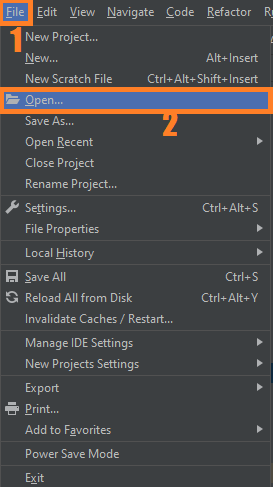# Live Sketch OpenCV Python With Source Code

## Live Sketch OpenCV Python With Source Code

The Live Sketch OpenCV Python was developed using Python OpenCV, In this Project we are going to make a Real-timelive Sketch making script using OpenCV in Python. OpenCV makes it very easy for us to work with images and videos on the computer.

A Live Sketch is an application which will show a live sketch of your webcam feed. In this project we’ll be using NumPy and OpenCV. We will also make use of Numpy and Matplotlib to make this live sketch app.

## What is OpenCV?

OpenCV (Open Source Computer Vision Library) is an open-source computer vision and machine learning software library. OpenCV was built to provide a common infrastructure for computer vision applications and to accelerate the use of machine perception in commercial products.

## What is NumPy?

NumPy is the fundamental package for scientific computing with Python. It contains among other things:

1. A powerful N-dimensional array object
3. Tools for integrating C/C++ and Fortran code
4. Useful linear algebra, Fourier transform, and random number capabilities Besides its obvious scientific uses.

By the way if you are new to python programming and you don’t know what would be the the Python IDE to use, I have here a list of Best Python IDE for Windows, Linux, Mac OS that will suit for you. I also have here How to Download and Install Latest Version of Python on Windows.

To start executing Live Sketch OpenCV Python With Source Code, make sure that you have installed Python 3.9 and PyCharm in your computer.

## Live Sketch OpenCV Python With Source Code : Steps on how to run the project

Time needed: 5 minutes

These are the steps on how to run Live Sketch OpenCV Python With Source Code

First, download the given source code below and unzip the source code.• Step 2: Import the project to your PyCharm IDE.• Step 3: Run the project.

last, run the project with the command “py main.py”## Installed Libraries

```import cv2 as cv
import numpy as np
from matplotlib import pyplot as plt```

## Complete Source Code

```import cv2 as cv
import numpy as np
from matplotlib import pyplot as plt

def sketch(image):
# Convert image to gray scale
img_gray = cv.cvtColor(image, cv.COLOR_BGR2GRAY)

# Clean up image using Gaussian Blur
img_gray_blur = cv.GaussianBlur(img_gray, (5, 5), 0)

# Extract Edges
canny_edges = cv.Canny(img_gray_blur, 30, 70)

# Do an invert binarize the image
ret, mask = cv.threshold(canny_edges, 120, 255, cv.THRESH_BINARY_INV)

def liveSketch():
cap = cv.VideoCapture(0)

while True:
cv.imshow("Live Sketch", sketch(frame))
if cv.waitKey(1) == 27:
break

cap.release()
cv.destroyAllWindows()

if __name__ == "__main__":
liveSketch()
```